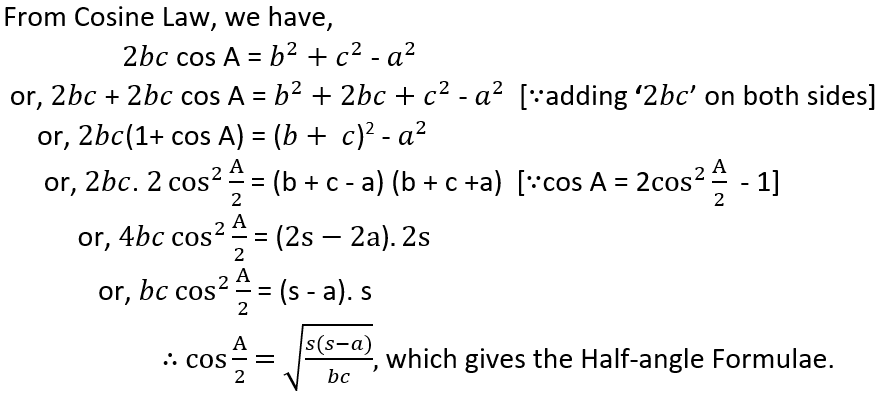# The Half-angle FormulaFor Cosine values,The other results of cosine values in Half-angle formula follow the same process.

For Sine valuesThe other results of sine value in Half-angle formula follow the same process.

For Tangent valuesThe other results of tangent values in Half-angle formula follow the same process.